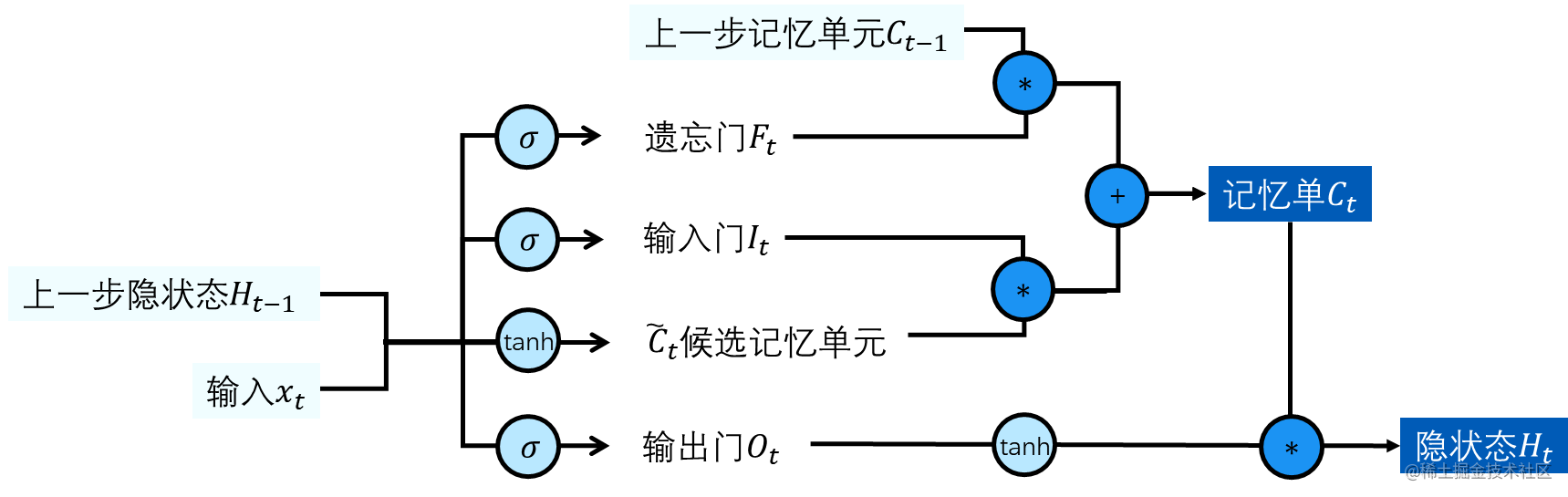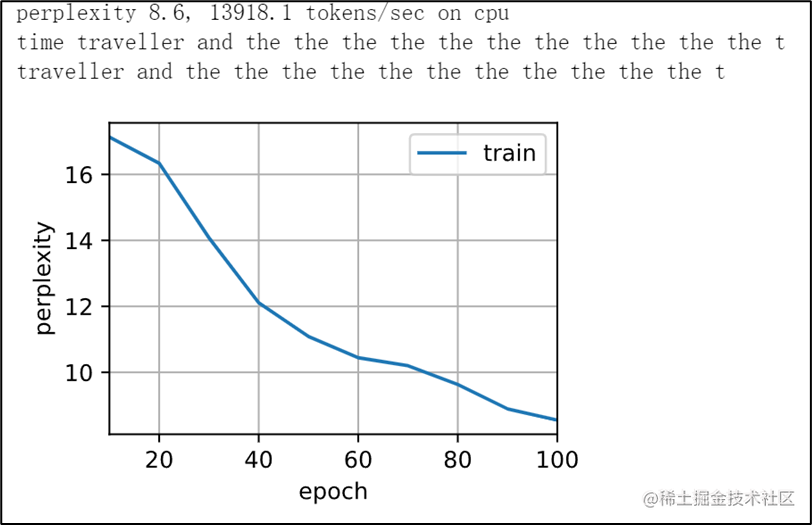# 公式程式碼都有了，速來學LSTM 長短期記憶網路

## theme: cyanosis

Long Short-Term Memory | MIT Press Journals & Magazine | IEEE Xplore

# 先來看一下公式• 每一步計算都需要三部分內容：

• 上一個時間步傳遞過來的記憶單元

• 上一個時間步步傳遞過來的隱狀態

• 本時間步的輸入
• 每一步計算的輸出都有兩部分內容：

• 本時間步的記憶單元

• 本時間步的隱藏狀態

• 淺藍色圓圈表示神經網路使用的啟用函式

• 深藍色圓圈表示運算過程

## 三門

LSTM有三個門，它分別是輸入門$\mathbf{I}_t$、忘記門$\mathbf{F}_t$和輸出門$\mathbf{O}_t$

\begin{aligned} &\mathbf{I}t = \sigma(\mathbf{X}_t \mathbf{W}{xi} + \mathbf{H}{t-1} \mathbf{W}{hi} + \mathbf{b}i),\ &\mathbf{F}_t = \sigma(\mathbf{X}_t \mathbf{W}{xf} + \mathbf{H}{t-1} \mathbf{W}{hf} + \mathbf{b}f),\ &\mathbf{O}_t = \sigma(\mathbf{X}_t \mathbf{W}{xo} + \mathbf{H}{t-1} \mathbf{W}{ho} + \mathbf{b}_o), \end{aligned}

• 其中輸入 $\mathbf{X}_t \in \mathbb{R}^{n \times d}$
• 前一時間步的隱藏狀態為 $\mathbf{H}_{t-1} \in \mathbb{R}^{n \times h}$。
• $t$時間步時， 輸入門$\mathbf{I}_t \in \mathbb{R}^{n \times h}$，遺忘門$\mathbf{F}_t \in \mathbb{R}^{n \times h}$，輸出門$\mathbf{O}_t \in \mathbb{R}^{n \times h}$。
• $\mathbf{W}{xi}, \mathbf{W}{xf}, \mathbf{W}{xo} \in \mathbb{R}^{d \times h}$ 和 $\mathbf{W}{hi}, \mathbf{W}{hf}, \mathbf{W}{ho} \in \mathbb{R}^{h \times h}$ 是權重引數
• $\mathbf{b}_i, \mathbf{b}_f, \mathbf{b}_o \in \mathbb{R}^{1 \times h}$ 是偏置引數。
• 啟用函式依舊使用sigmoid

\begin{aligned} &\mathbf{I}t = \sigma([\mathbf{X}_t ,\mathbf{H}{t-1}] \mathbf{W}{i} + \mathbf{b}_i),\ &\mathbf{F}_t = \sigma([\mathbf{X}_t ,\mathbf{H}{t-1}] \mathbf{W}{f} + \mathbf{b}_f),\ &\mathbf{O}_t = \sigma([\mathbf{X}_t ,\mathbf{H}{t-1}] \mathbf{W}_{o} + \mathbf{b}_o), \end{aligned}

## 候選記憶單元

$$\tilde{\mathbf{C}}t = \text{tanh}(\mathbf{X}_t \mathbf{W}{xc} + \mathbf{H}{t-1} \mathbf{W}{hc} + \mathbf{b}_c)$$

• $\mathbf{W}{xc} \in \mathbb{R}^{d \times h}$ 和 $\mathbf{W}{hc} \in \mathbb{R}^{h \times h}$ 是權重引數。
• $\mathbf{b}_c \in \mathbb{R}^{1 \times h}$ 是偏置引數。
• 候選記憶單元使用的啟用函式是tanh。

## 記憶單元

$$\mathbf{C}t = \mathbf{F}_t \odot \mathbf{C}{t-1} + \mathbf{I}_t \odot \tilde{\mathbf{C}}_t$$

• $\odot$在這裡的意思是按矩陣元素位置相乘，不是做普通的矩陣運算。

• 如果遺忘門為 $1$ 且輸入門為 $0$，則過去的記憶單元 $\mathbf{C}_{t-1}$ 將隨時間被儲存並傳遞到當前時間步。
• 如果遺忘門為 $0$ 且輸入門為 $1$，則過去的記憶單元 $\mathbf{C}_{t-1}$ 被丟棄掉，僅使用當前的候選記憶單元$\tilde{\mathbf{C}}_t$。

## 隱藏單元

$$\mathbf{H}_t = \mathbf{O}_t \odot \tanh(\mathbf{C}_t)$$

• 輸出門接近 $1$，我們就能夠把我們的記憶單元資訊傳遞下去。
• 輸出門接近 $0$，我們只保留儲存單元內的所有資訊。

# 程式碼

python import torch from torch import nn from d2l import torch as d2l from torch.nn import functional as F 導包啊導包，這個不用解釋了吧。

py train_iter, vocab = d2l.load_data_time_machine(batch_size, num_steps) batch_size, num_steps = 32, 35 這裡直接使用d2l載入資料集。

python class LSTMModel(nn.Module): def init(self, lstm_layer, vocab_size, kwargs): super(LSTMModel, self).init(kwargs) self.lstm = lstm_layer self.vocab_size = vocab_size self.num_hiddens = self.lstm.hidden_size if not self.lstm.bidirectional: self.num_directions = 1 self.linear = nn.Linear(self.num_hiddens, self.vocab_size) else: self.num_directions = 2 self.linear = nn.Linear(self.num_hiddens * 2, self.vocab_size)

def forward(self, inputs, state):
X = F.one_hot(inputs.T.long(), self.vocab_size)
X = X.to(torch.float32)
Y, state = self.lstm(X, state)
output = self.linear(Y.reshape((-1, Y.shape[-1])))
return output, state

def begin_state(self, device, batch_size=1):
return (torch.zeros((
self.num_directions * self.lstm.num_layers,
batch_size, self.num_hiddens), device=device),
torch.zeros((
self.num_directions * self.lstm.num_layers,
batch_size, self.num_hiddens), device=device))




• __init__初始化這個類，這個類是繼承了nn.Module的。

• self.lstm設定計算層是LSTM層

• self.vocab_size設定字典的大小，這裡大小是28，因為為了方便演示，我們這裡使用的是字母進行分詞，所以其中只有a\~z26個字母外加<unk>（空格和unknown）。

• self.num_hiddens設定隱藏層的大小。 > 可能這裡會導致迷惑，我剛開始看的時候也有一瞬間的迷惑。普通rnn的隱藏層，不是說其他的就是隱狀態了嗎？
> 不是這樣的，普通rnn是普通隱藏層，現在的GRU。LSTM是含有隱狀態的隱藏層。所以還是隱藏層。
• if-else語句是設定LSTM單雙向的，畢竟還有雙向LSTM這種東西的存在。
• forward定義前向傳播網路。

也就是描述計算過程。

• 這裡首先是將輸入轉化為對應的one-hot向量，再將其型別轉化為float。

• Ystate是計算隱狀態的，這裡Y是輸出全部的隱狀態，state是輸出最後一個時間步的隱狀態。注意 在這裡Y不是 輸出。

• output是用於儲存輸出的。
• begin_state是進行初始化。

這裡是return了好長一個句子。可以拆解開看一下子。這裡是初始化為0張量，初始化位置device=device由你傳入的位置決定是CPU還是GPU。這裡和普通RNN的區別在於普通RNN和GRU不同，二者只需要返回一個張量即可，但LSTM這裡是一個元組裡兩個張量。

python vocab_size, num_hiddens, device = len(vocab), 256, d2l.try_gpu() num_epochs, lr = 100, 1 num_inputs = vocab_size lstm_layer = nn.LSTM(num_inputs, num_hiddens) model = LSTMModel(lstm_layer, len(vocab)) model = model.to(device) d2l.train_ch8(model, train_iter, vocab, lr, num_epochs, device)

• 第一句和第二句分別是設定詞典長度、隱藏層大小、執行在CPU還是GPU上、訓練epoch數量、學習率。

• 設定lstm使用pytorch自帶的nn.LSTM

• 模型使用我們的那個類，並且將其放到對應的裝置上執行。
• 最後一句就是預測訓練的過程。